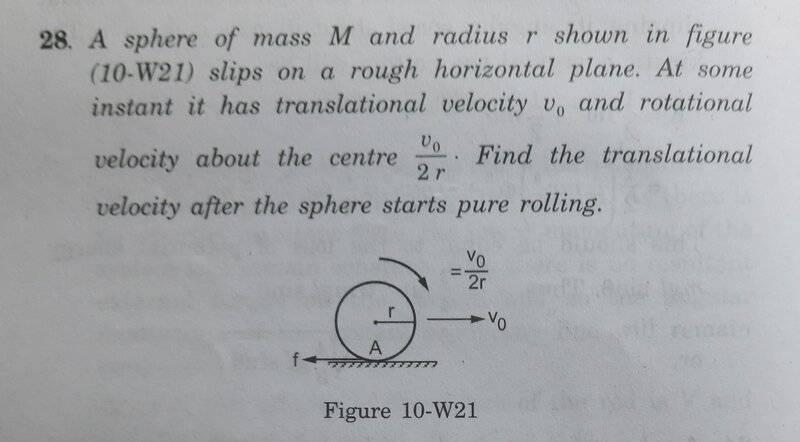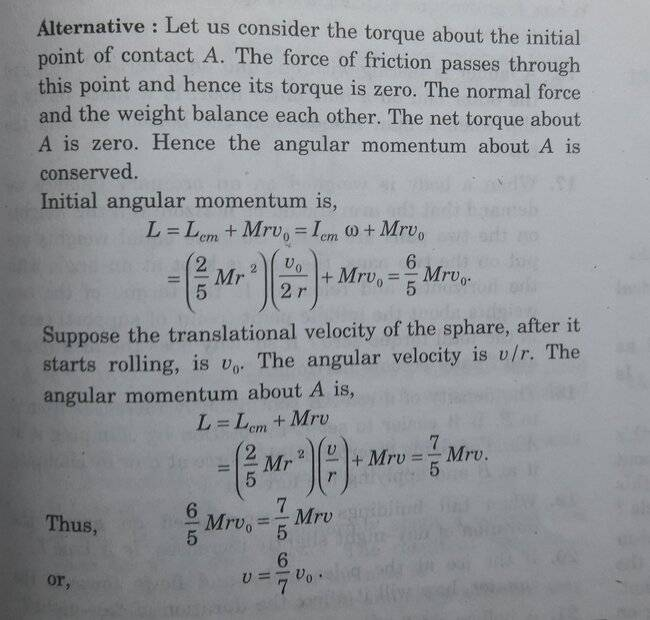# Doubt regarding the terms used in the solution

• B
tbn032In the solution, the term Lcm and Icm is used. Explain the meaning of these terms? I think cm stands for centre of mass. why that is used in the subscript?does the term angular momentum from the centre of mass of the sphere makes sense? Is the term Lcm and Icm stand for angular momentum of the sphere from the axis passing through the centre of mass of the sphere and moment of inertia of sphere from the axis passing through the centre of mass of the sphere respectively.

Homework Helper
Gold Member
2022 Award
View attachment 313101 Is the term Lcm and Icm stand for angular momentum of the sphere from the axis passing through the centre of mass of the sphere and moment of inertia of sphere from the axis passing through the centre of mass of the sphere respectively.
Yes.

tbn032
Also in the solution it is written "suppose the translational velocity of the sphere after it start rolling,is vο"is this a misprint ?, and it should have been written v instead of vο.